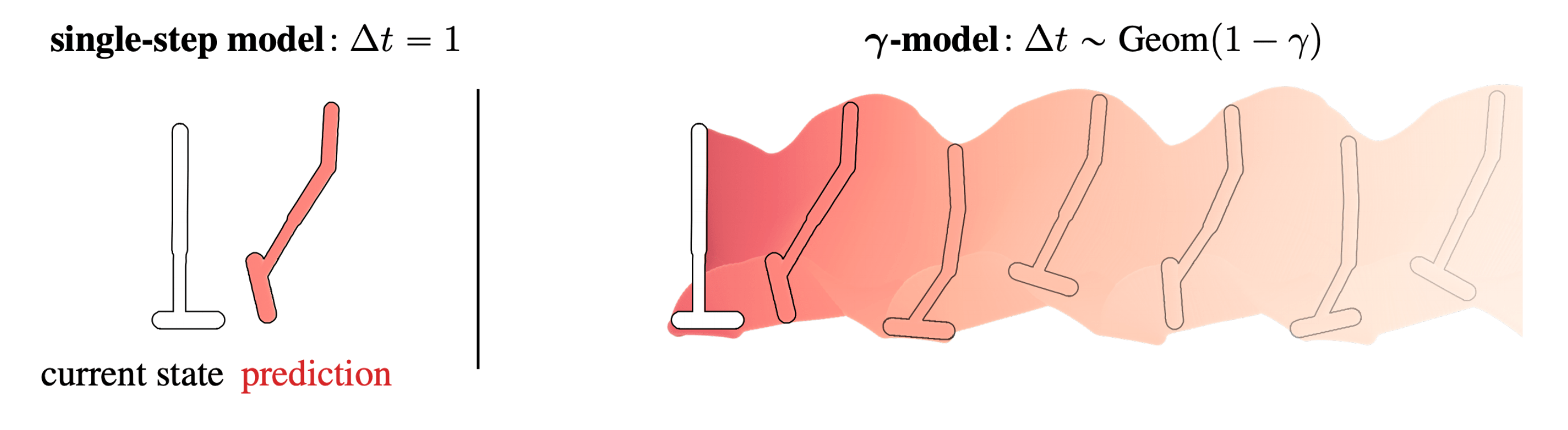# The Successor Representation, $\gamma$-Models, and Infinite-Horizon Prediction — The Berkeley Artificial Intelligence Research BlogThe Successor Representation, Gamma-Models, and Infinite-Horizon Prediction Standard single-step models have a horizon of one. This post describes a method for training predictive dynamics models in continuous state spaces with an infinite, probabilistic horizon. Reinforcement learning algorithms are frequently categorized by whether they predict future states at any point in their decision-making process. Those that…

The Successor Representation, $\gamma$-Models, and Infinite-Horizon Prediction — The Berkeley Artificial Intelligence Research Blog